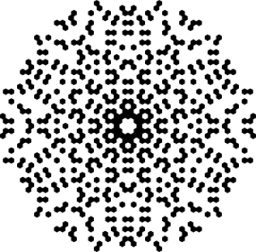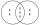# Reminder and quotient

There are given numbers A = 135, B = 315. Find the smallest natural number R greater than 1 so that the proportions R:A, R:B are with the remainder 1.

R =  946

### Step-by-step explanation:Did you find an error or inaccuracy? Feel free to write us. Thank you!Math student
Calculatin is all wrong.
Smallest number after 1 is 2 .
And 2 also give me the remainder 1.
135/2 and 315/2 calculate yourself.Dr Math

2:135 is 0 and reminder 2 !!!Tips to related online calculators
Do you want to calculate least common multiple two or more numbers?
Need help to calculate sum, simplify or multiply fractions? Try our fraction calculator.
Do you want to perform natural numbers division - find the quotient and remainder?

## Related math problems and questions:

• Reminder and quotientThere are given the number C = 281, D = 201. Find the highest natural number S so that the C:S, D:S are with the remainder of 1,
• Solve 2Solve integer equation: a +b+c =30 a, b, c = can be odd natural number from this set (1,3,5,7,9,11,13,15)
• Unknown integerFind the smallest integer: divided by 2, the remainder is 1. divided by 3, the remainder is 2. divided by 4, the remainder is 3. ... divided by eight, the remainder is 7, divided by 9 the remainder is 8.
• LCM of two numberFind the smallest multiple of 63 and 147
• AthletesAthletes at the stadium could enter two-steps, three-steps, four-steps, five-steps, six-steps. There were more than 100 but less than 200. How many athletes were there?
• Magic numberThe number 135 split to two addends so that one addend was 30 greater than 2/5 the addend.
• LCD 2The least common denominator of 2/5, 1/2, and 3/4
• Prime divisorsFind 2/3 of the sum's ratio and the product of all prime divisors of the number 120.
• Lcm simpleFind least common multiple of this two numbers: 140 175.
• Lcm of three numbersWhat is the Lcm of 120 15 and 5
• Divisible by 5How many three-digit odd numbers divisible by 5, which are in place ten's number 3?
• Counting numberWhat is the smallest counting number divisible by 2,5,7,8 and 15?
• TissuesThe store got three kinds of tissues - 132 children, 156 women and 204 men. Tissues each species were packed into boxes after the number of pieces the same for all three types (and greatest). Determine the number, if you know that every box has more than
• Coloured numbersMussel wrote four different natural numbers with coloured markers: red, blue, green and yellow. When the red number divides by blue, it gets the green number as an incomplete proportion, and yellow represents the remainder after this division. When it div
• Lcm 2Create the smallest possible number that is divisible by numbers 5,8,9,4,3
• A number 5A number is divisible by 24, 25, and 120 if it is increased by 20. Find the number.
• Find unknown numberWhat is the number between 50 and 55 that is divisible by 2,3,6,9?